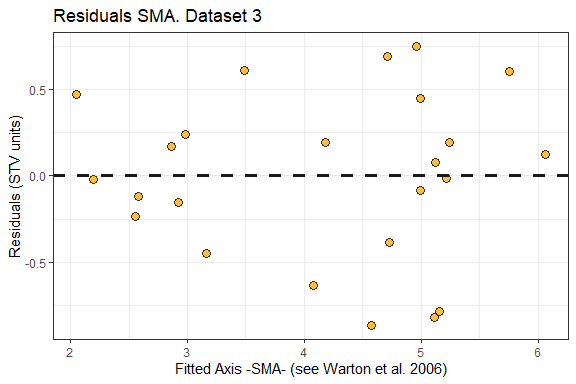# Modified ALCC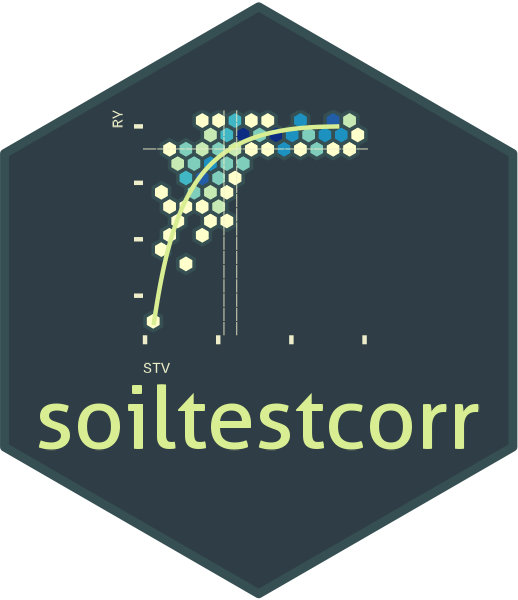## Description

This tutorial is intended to show how to deploy the mod_alcc() function for estimating critical soil test values using the Modified Arcsine-Log Calibration Curve, originally introduced by Dyson & Conyers (2013) and modified by Correndo et al. (2017). This function produces the estimation of critical soil test values (CSTV) for a target relative yield (ry) with confidence intervals at adjustable confidence levels. Behind the scenes, the mod_alcc() is based on the standardized major axis (SMA), a bivariate regression model that assumes that both axis are random variables (Warton et al., 2006).

## General Instructions

1. Load your dataframe with soil test value (stv) and relative yield (ry) data.
2. Specify the following arguments into the function mod_alcc()
1. data (optional)

2. stv (soil test value) and ry (relative yield) columns or vectors,

3. target of relative yield (e.g. 90%),

4. desired confidence level (e.g. 0.95 for 1 - alpha(0.05)).

• Used for the estimation of critical soil test value (CSTV) lower and upper limits.
5. tidy TRUE (produces a data.frame with results) or FALSE-default- (store results as list),

6. plot TRUE (produces a ggplot as main output) or FALSE (default; no plot, only results as data.frame or list)

3. Run and check results.
4. Check residuals plot, and warnings related to potential leverage points.
5. Adjust curve plots as desired with additional ggplot2 functions.

# Tutorial

library(soiltestcorr)

Suggested packages

# Install if needed
library(ggplot2) # Plots
library(dplyr) # Data wrangling
library(tidyr) # Data wrangling
library(purrr) # Mapping

This is a basic example using three different datasets:

# Native fake dataset from soiltestcorr package
corr_df <- soiltestcorr::freitas1966

# Fit mod_alcc()

## 1. Individual fits

RY target = 90%, confidence level = 0.95, replace with your desired values

### 1.1. tidy = FALSE

It returns a LIST (may be more efficient for multiple fits at once)

# Using dataframe argument, tidy = FALSE -> return a LIST
mod_alcc(data = corr_df, ry = RY, stv = STK, target=90, confidence = 0.95,
tidy = TRUE)
#> Warning: One or more original RY values exceeded 100%. All RY values greater
#>           than 100% have been capped to 100%.
#> Warning: 2 STV points exceeded the CSTV for 100% of RY.
#>   Risk of leverage. You may consider a sensitivity analysis by removing extreme points,
#>   re-run the mod_alcc(), and check results.
#> Warning: 2 STV points exceeded two-times (2x)
#>   the CSTV for 90% of RY. Risk of leverage. You may consider a sensitivity analysis by
#>   removing extreme points, re-run the mod_alcc(), and check results.
#> # A tibble: 1 × 18
#>       n     r RMSE_…¹ AIC_a…² AIC_sma BIC_sma p_value confi…³ target  CSTV    LL
#>   <int> <dbl>   <dbl>   <dbl>   <dbl>   <dbl>   <dbl>   <dbl>  <dbl> <dbl> <dbl>
#> 1    24 0.728    10.3    186.    17.0    22.9 5.57e-5    0.95     90  76.9  62.3
#> # … with 7 more variables: UL <dbl>, CSTV90 <dbl>, n.90x2 <int>, CSTV100 <dbl>,
#> #   n.100 <int>, Curve <list>, SMA <list>, and abbreviated variable names
#> #   ¹​RMSE_alcc, ²​AIC_alcc, ³​confidence

### 1.2. tidy = TRUE

It returns a data.frame (more organized results)

# Using dataframe argument, tidy = FALSE -> return a LIST
mod_alcc(data = corr_df, ry = RY, stv = STK, target=90, confidence = 0.95, tidy = TRUE)
#> Warning: One or more original RY values exceeded 100%. All RY values greater
#>           than 100% have been capped to 100%.
#> Warning: 2 STV points exceeded the CSTV for 100% of RY.
#>   Risk of leverage. You may consider a sensitivity analysis by removing extreme points,
#>   re-run the mod_alcc(), and check results.
#> Warning: 2 STV points exceeded two-times (2x)
#>   the CSTV for 90% of RY. Risk of leverage. You may consider a sensitivity analysis by
#>   removing extreme points, re-run the mod_alcc(), and check results.
#> # A tibble: 1 × 18
#>       n     r RMSE_…¹ AIC_a…² AIC_sma BIC_sma p_value confi…³ target  CSTV    LL
#>   <int> <dbl>   <dbl>   <dbl>   <dbl>   <dbl>   <dbl>   <dbl>  <dbl> <dbl> <dbl>
#> 1    24 0.728    10.3    186.    17.0    22.9 5.57e-5    0.95     90  76.9  62.3
#> # … with 7 more variables: UL <dbl>, CSTV90 <dbl>, n.90x2 <int>, CSTV100 <dbl>,
#> #   n.100 <int>, Curve <list>, SMA <list>, and abbreviated variable names
#> #   ¹​RMSE_alcc, ²​AIC_alcc, ³​confidence

### 1.3. Alternative using the vectors

You can call stv and ry vectors using the $. The tidy argument still applies for controlling the output type fit_vectors_tidy <- mod_alcc(ry = corr_df$RY,
stv = corr_df$STK, target = 90, confidence = 0.95) #> Warning: One or more original RY values exceeded 100%. All RY values greater #> than 100% have been capped to 100%. #> Warning: 2 STV points exceeded the CSTV for 100% of RY. #> Risk of leverage. You may consider a sensitivity analysis by removing extreme points, #> re-run the mod_alcc(), and check results. #> Warning: 2 STV points exceeded two-times (2x) #> the CSTV for 90% of RY. Risk of leverage. You may consider a sensitivity analysis by #> removing extreme points, re-run the mod_alcc(), and check results. fit_vectors_list <- mod_alcc(ry = corr_df$RY,
stv = corr_df$STK, target = 90, confidence = 0.95, tidy = FALSE) #> Warning: One or more original RY values exceeded 100%. All RY values greater #> than 100% have been capped to 100%. #> Warning: 2 STV points exceeded the CSTV for 100% of RY. #> Risk of leverage. You may consider a sensitivity analysis by removing extreme points, #> re-run the mod_alcc(), and check results. #> Warning: 2 STV points exceeded two-times (2x) #> the CSTV for 90% of RY. Risk of leverage. You may consider a sensitivity analysis by #> removing extreme points, re-run the mod_alcc(), and check results. ## 2. Multiple fits at once # Example 1. Fake dataset manually created data_1 <- data.frame("RY" = c(65,80,85,88,90,94,93,96,97,95,98,100,99,99,100), "STV" = c(1,2,3,4,5,6,7,8,9,10,11,12,13,14,15)) # Example 2. Native fake dataset from soiltestcorr package data_2 <- soiltestcorr::data_test # Example 3. Native dataset from soiltestcorr package, Freitas et al. (1966), used by Cate & Nelson (1971) data_3 <- soiltestcorr::freitas1966 %>% rename(STV = STK) data.all <- bind_rows(data_1, data_2, data_3, .id = "id") Note: the stv column needs to have the same name for all datasets if binding rows. ### 2.1. Using map()  # Run multiple examples at once with purrr::map() data.all %>% nest(data = c("STV", "RY")) %>% mutate(model = map(data, ~ mod_alcc(stv = .$STV, ry = .$RY, target = 90))) %>% unnest(model) #> Warning: There were 9 warnings in mutate(). #> The first warning was: #> ℹ In argument: model = map(data, ~mod_alcc(stv = .$STV, ry = .$RY, target = #> 90)). #> Caused by warning: #> ! You have not specified the confidence level. #> Please, modify if your desired confidence is different than the default (0.95) #> ℹ Run dplyr::last_dplyr_warnings() to see the 8 remaining warnings. #> # A tibble: 3 × 20 #> id data n r RMSE_alcc AIC_alcc AIC_sma BIC_sma p_value confi…¹ #> <chr> <list> <int> <dbl> <dbl> <dbl> <dbl> <dbl> <dbl> <dbl> #> 1 1 <tibble> 15 0.968 1.45 59.6 -23.5 -20.0 3.30e- 9 0.95 #> 2 2 <tibble> 137 0.716 10.5 1038. -1.04 13.6 7.31e-23 0.95 #> 3 3 <tibble> 24 0.728 10.3 186. 17.0 22.9 5.57e- 5 0.95 #> # … with 10 more variables: target <dbl>, CSTV <dbl>, LL <dbl>, UL <dbl>, #> # CSTV90 <dbl>, n.90x2 <int>, CSTV100 <dbl>, n.100 <int>, Curve <list>, #> # SMA <list>, and abbreviated variable name ¹​confidence Note: the output table is still generated despite warnings regarding confidence level and leverage points. ### 2.2. Using group_modify() Alternatively, with group_modify, nested data is not required. However, it still requires a grouping variable (in this case, id) to identify each dataset. group_map() may also be used, though list_rbind() is required to return a tidy data frame of the model results instead of a list.  data.all %>% group_by(id) %>% group_modify(~ mod_alcc(data = ., STV, RY, target = 90, confidence = 0.95)) #> Warning: One or more original RY values exceeded 100%. All RY values greater #> than 100% have been capped to 100%. #> # A tibble: 3 × 19 #> # Groups: id  #> id n r RMSE_alcc AIC_alcc AIC_sma BIC_sma p_value confid…¹ target #> <chr> <int> <dbl> <dbl> <dbl> <dbl> <dbl> <dbl> <dbl> <dbl> #> 1 1 15 0.892 1.79 66.1 -82.7 -79.1 7.84e- 6 0.95 90 #> 2 2 137 0.569 11.6 1067. -290. -275. 4.14e-13 0.95 90 #> 3 3 24 0.708 20.0 218. -5.01 0.885 1.10e- 4 0.95 90 #> # … with 9 more variables: CSTV <dbl>, LL <dbl>, UL <dbl>, CSTV90 <dbl>, #> # n.90x2 <int>, CSTV100 <dbl>, n.100 <int>, Curve <list>, SMA <list>, and #> # abbreviated variable name ¹​confidence ## 3. Bootstrapping Bootstrapping is a suitable method for obtaining confidence intervals for parameters or derived quantities. Bootstrapping is a resampling technique (with replacement) that draws samples from the original data with the same size. If you have groups within your data, you can specify grouping variables as arguments in order to maintain, within each resample, the same proportion of observations than in the original dataset. This function returns a table with as many rows as the resampling size (n) containing the results for each resample. set.seed(123) boot_alcc <- boot_mod_alcc(data = corr_df, stv = STK, ry = RY, target = 90, n = 500) #> Warning: There were 1332 warnings in dplyr::mutate(). #> The first warning was: #> ℹ In argument: model = map(...). #> ℹ In group 1: boot_id = 1. #> Caused by warning: #> ! One or more original RY values exceeded 100%. All RY values greater #> than 100% have been capped to 100%. #> ℹ Run dplyr::last_dplyr_warnings() to see the 1331 remaining warnings. boot_alcc %>% head(n = 5) #> # A tibble: 5 × 14 #> boot_id n r RMSE_alcc AIC_alcc AIC_sma BIC_sma p_value target CSTV #> <dbl> <int> <dbl> <dbl> <dbl> <dbl> <dbl> <dbl> <dbl> <dbl> #> 1 1 24 0.508 12.4 195. 11.9 17.7 0.0112 90 81.9 #> 2 2 24 0.750 9.50 182. 7.68 13.6 0.0000240 90 61.8 #> 3 3 24 0.625 10.6 188. 10.6 16.5 0.00109 90 86.6 #> 4 4 24 0.688 8.96 179. 16.1 22.0 0.000204 90 72.4 #> 5 5 24 0.593 9.74 183. 13.3 19.2 0.00225 90 80.9 #> # … with 4 more variables: CSTV90 <dbl>, n.90x2 <int>, CSTV100 <dbl>, #> # n.100 <int> # CSTV Confidence Interval quantile(boot_alcc$CSTV, probs = c(0.025, 0.5, 0.975))
#>     2.5%      50%    97.5%
#> 63.70241 77.17436 93.90476

# Plot
boot_alcc %>%
ggplot2::ggplot(aes(x = CSTV))+
geom_histogram(color = "grey25", fill = "steelblue", bins = 10)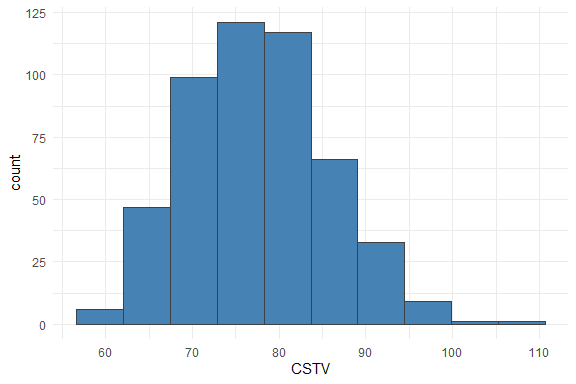## 4. Plots

### 4.1. Calibration Curve

We can generate a ggplot with the same mod_alcc() function. We just need to specify the argument plot = TRUE.

plt_alcc <- mod_alcc(data = corr_df,
ry = RY,
stv = STK,
target = 95,
plot = TRUE)

plt_alcc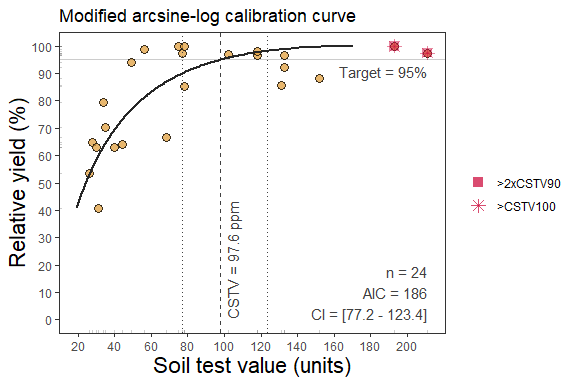### 4.2. Fine-tune the plots

As ggplot object, plots can be adjusted in several ways, such as modifying titles and axis scales.

plt_alcc +
# Main title
ggtitle("My own plot title")+
# Axis titles
labs(x = "Soil Test K (ppm)",
y = "Cotton RY(%)") +
# Axis scales
scale_x_continuous(limits = c(20,220),
breaks = seq(0,220, by = 25)) +
# Axis limits
scale_y_continuous(limits = c(30,100),
breaks = seq(20,100, by = 20))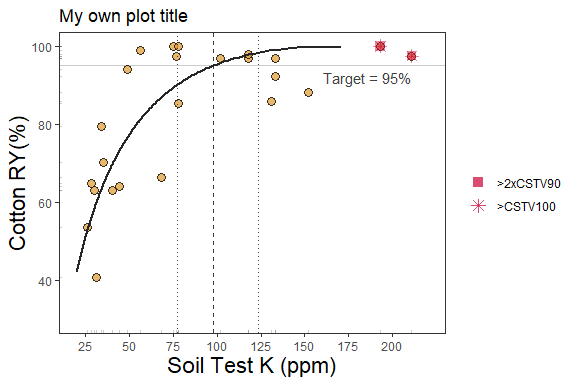### 3.2. SMA regression

Behind the scenes, the mod_alcc() runs a Standardized Major Axis regression (SMA).

We can extract the SMA fit and also check the residuals of this model out as follows:

fit_3 <- mod_alcc(data = corr_df, ry = RY, stv = STK, target = 90)
# Extract SMA regression fit and residuals from fit_3 (data_3, (Freitas et al., 1966))
SMA_freitas_1966 <- fit_3\$SMA %>% as.data.frame()

SMA_freitas_1966 %>%
ggplot(aes(x = arc_RY, y = ln_STV))+
ggtitle("SMA Regression. Dataset 3")+
geom_point(shape=21, fill = "orange", size = 3, alpha = 0.75)+
#SMA Line
geom_path(aes(x=arc_RY, y = SMA_line, linetype = "SMA_fit"), linewidth = 1.5, col = "grey25")+
scale_linetype_manual(name="", values = c("solid"))+
#Critical value
geom_vline(xintercept = 0, col = "grey10", size = 1.25, linetype = "dashed")+
theme_bw()+
# Axis titles
labs(y = "ln_STV", y = "asin(sqrt(RY))-centered")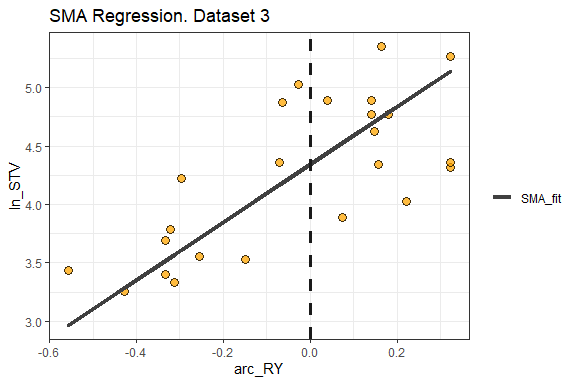### 3.3. SMA residuals


# Residuals plot
SMA_freitas_1966 %>%
ggplot(aes(x = fitted_axis, y = residuals))+
ggtitle("Residuals SMA. Dataset 3")+
geom_point(shape=21, fill = "orange", size = 3, alpha = 0.75)+
geom_hline(yintercept = 0, col = "grey10", linewidth = 1.25, linetype = "dashed")+
theme_bw()+
# Axis titles
labs(x = "Fitted Axis -SMA- (see Warton et al. 2006)", y = "Residuals (STV units)")